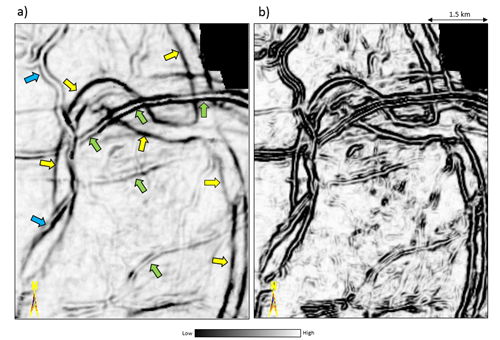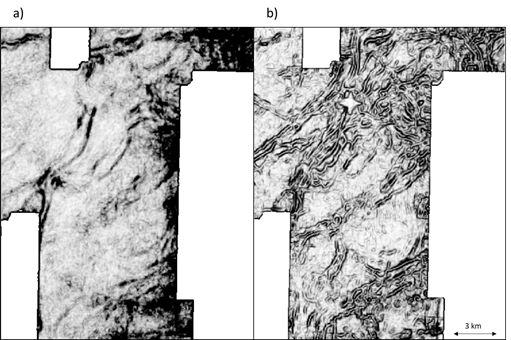# Sobel-filter

## For a flat photograph containing pixels of a given amplitude aligned along the x and y axes, the classical Sobel-filtered image, is simply obtained by running a process equivalent to the square-root of the sum of the squares of derivatives of the amplitude in the x and the y directions.

Unlike a photograph, seismic images have a third dimension. A similar process (equivalent to the square-root of the sum of the squares of derivatives of the amplitude in the inline and crossline directions along structural dip) normalized by the RMS amplitude in the window of application to account for structural dip can be run on the seismic data. This normalization will enhance lower amplitude edges when applied to a coherence input volume.Stratal-slices at a level close to a horizon picked at t=1020 ms through (a) energy ratio coherence volume computed from the seismic amplitude, and (b) Sobel-filter similarity computed from the coherence volume shown in (a).  Notice the clarity with which the edges of channels seen in (a) are seen in (b).Time slice at t=1020 ms from (a) coherence volume computed using the energy ratio algorithm, and (b) Sobel-filter run on the coherence shown in (a).  Notice the clarity with which the edges of channels seen in (a) are seen in (b).